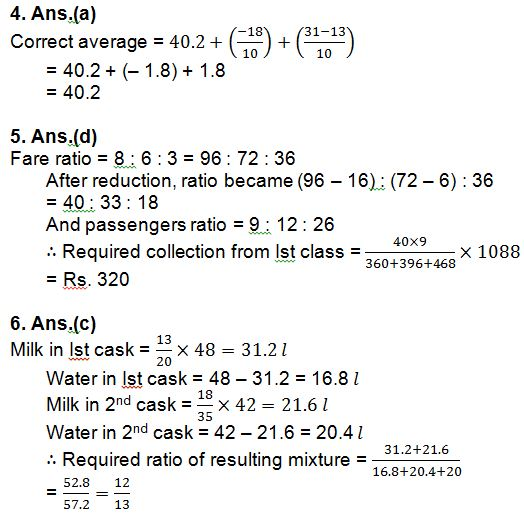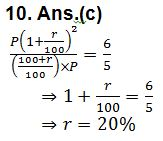# Quant Quiz (Misc) 15th September 2018

Q1. Sum of two numbers is equal to sum of square of 11 and cube of 9. Larger number is less than square of 25. What is the value of the sum of twice of 24 percent of the smaller number and half of the larger number?

(a) 415

(b) 400

(c) 410

(d) 425

(e) None of these

Q2. Village A has a population of 6800, which is decreasing at the rate of 120 per year. Village B has a population of 4200, which is increasing at the rate of 80 per year. In how many years will the population of the two village be equal?

(a) 9 yr

(b) 11 yr

(c) 13 yr

(d) 16 yr

(e) None of these

Q3. 40% of the students in a college play Basket ball, 34% of the students play Tennis and the number of students who play both the games is 234. The number of students who play neither Basket ball nor Tennis is 52%. Determine the student population in the college.

(a) 800

(b) 1050

(c) 900

(d) 850

(e) None of these

Q4. The average of 10 numbers is 40.2 Later it is found that two numbers have been wrongly added. The first is 18 greater than the actual number and the second number added is 13 instead of 31. Find the correct average.

(a) 40.2

(b) 40.4

(c) 40.6

(d) 40.8

(e) None of these

Q5. Railway fares of Ist, 2nd and 3rd classes between two stations were in the ratio of 8 : 6 : 3. The fares of 1st and 2nd class were subsequently reduced by 1/6 and 1/12, respectively. If during a year, the ratio between the passengers of Ist, 2nd and 3rd classes was 9 : 12 : 26 and total amount collected after reduction in fares by the sale of tickets was Rs. 1088, then find the collection from the passengers of 1st class.

(a) Rs. 260

(b) Rs. 280

(c) Rs. 300

(d) Rs. 320

(e) None of these

Q6. Two casks of 48 litres and 42 litres are filled with mixtures of milk and water; the proportions in the two casks being respectively, 13 : 7 and 18 : 17. If the contents of the two casks be mixed, and 20 litres of water be added to the whole, what will be the proportion of milk and water in the resulting mixture?

(a) 5 : 12

(b) 7 : 13

(c) 12 : 13

(d) 8 : 15

(e) None of these

Q7. Anu is a working partner and Bimla is a sleeping partner in a business. Anu puts in Rs. 5000 and Bimla puts in Rs. 6000. Anu receives 12.5% of the profit for managing the business and the rest is divided in proportion to their capital. What does each get out of a profit of Rs. 880?

(a) Rs. 400 and Rs. 480

(b) Rs. 460 and Rs. 420

(c) Rs. 450 and Rs. 430

(d) Rs. 470 and Rs. 410

(e) None of these

Q8. A manufacturer of a certain item can sell all he can produce at the selling price of Rs. 60 each. It costs him Rs. 40 in materials and labour to produce each item and he has overhead expenses of Rs. 3000 per week in order to operate that plant. The number of units he should produce and sell in order to make a profit of at least Rs. 1000 per week is –

(a) 250

(b) 300

(c) 400

(d) 200

(e) None of these

Q9. A horse and a carriage together cost Rs. 8000. If by selling the horse at a profit of 10% and the carriage at a loss of 10% a total profit of 2.5% is made, then what is the cost price of the horse?

(a) Rs. 3000

(b) Rs. 3500

(c) Rs. 4000

(d) Rs. 5000

(e) None of these

Q10. The ratio of the amount for two years under CI annually and for one year under SI is 6 : 5. When the rate of interest is same, then the value of the rate of interest is

(a) 12.5%

(b) 18%

(c) 20%

(d) 16.66%

(e) None of these

## SolutionSubscribe Our Youtube Channel click here

Mail us at:- ambitiousbaba1@gmail.com

Join Telegram study group: Click here